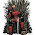## Tuesday, December 18, 2012

### NFL Picks Contest - Results and Standings

Here are the results and updated standings in the NFL Picks Contest

lightning- Houston (W 29-17) = 22 pts.
TwoMinuteWarning- Cincinnati (W 34-13) = 31pts.
Grange95-  Seattle (W 50-17) = 43 pts.
ohcowboy12go- New England (L 34-41) = (7 pts.)
Full House- New England (L 34-41) = (7 pts.)
Music City Momma- Seattle (W 50-17) = 43 pts.
Coach-  Detroit (L 10-38) = (28 pts.)
Waffles- Cincinnati (W 34-13) = 31 pts.
MOJO-  Houston (W 29-17) = 22 pts.
ggrouchie- Detroit (L 10-38) = (28 pts.)
Vegas Vic-  Seattle (W 50-17) = 43 pts.
Captain Crunch- Miami (W 24-3) = 31 pts.

Here are the current standings;

MOJO - 40+19+22+13+16+18+24+68+22 = 242 pts.
Grange95 - 20+19+22+13+14+40+24+23+43 = 218
Vegas Vic - 16+19+22+13+16+40+15+23+43 = 207
Music City Momma - 17+19+24+5+17+34+24+24+43 =  207
TwoMinuteWarning - 13+19+22+16+31+18+19+23+31 = 192
lightning - 13+19+24+13+16+18+24+24+22 = 173
Captain Crunch - 13+11+18+16+31+18+24-(2)+31 = 160
Coach - 20+19+22+13+16+40+17+38-(28) = 157
grrouchie - 14+48+20+5+16+40+17+23-(28) = 155
ohcowboy12go - 13+19+22+5+13+24+23-(7)=112
Waffles - 15+11+22+16+14+0+24-(28)+31 =105
Full House - 20+19+22-(34)+16+18+17+24-(7) =95

Congratulations to Grange, Music City Momma, and Vegas Vic for leading the way this week!

1.1.